Lesson 1

23.1.1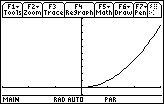The values of t are restricted to nonnegative values and because x = t the values of x are also restricted to those values.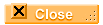23.1.2   Swapping x and y produces the inverse relation whose graph is the original graph reflected across the line y = x.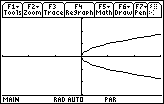23.1.3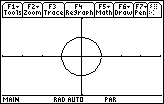[0, 2] x [-4, 4] x [-2, 2]

The first interval given in the window coordinates above represents t-values, the second and third intervals represent x and y-values, respectively.23.1.4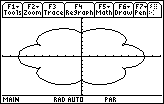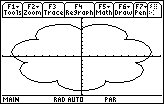x = 7sin t - sin 7t y = 7cos t - cos 7t x = 8sin t - sin 8t y = 8cos t - cos 8t

The number of petals is N - 1 in the epicycloid

x = N sin t - sin N t

y = N cos t - cos N tLesson 2

23.2.1 dydx(0.5) = 2.08583, which agrees with the value found using the "Derivative" feature of the Graph screen.23.2.2   The slope of the tangent line when t = 2 is dydx(2)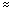0.64209323.2.3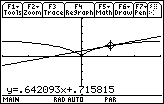The window shown is [-,] x [-3, 3] x [-5, 5].

The equation of the tangent to the curve when t = 2 is y0.642093x + 0.715815.Lesson 3

23.3.1 The arc length is 3/2 units.Self Test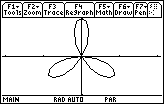1/2

7/43.34122 units